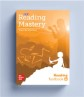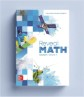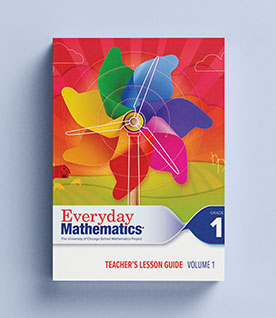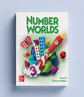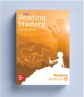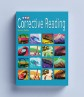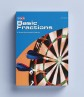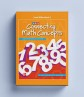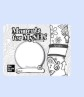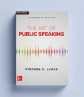## Our Principles# Problem Solver II

This series of six books guides students through step-by-step instruction in mathematical problem solving. Ten valuable problem-solving strategies are introduced and students learn how to apply each strategy using a 4-step method.

## Program Details

Problem Solver II builds on the concepts introduced in The Problem Solver. Problem Solver II offers hundreds of new problems that can be integrated into any math curriculum, and uses the same ten strategies and four-step method of problem solving. All problems are keyed to math strands and skills, thus allowing teachers to customize the program to meet the needs of students.

Features:

• Easy-to use format demonstrates how to guide students through the problem-solving process
• Introduces and explains 10 widely accepted problem-solving strategies that can be applied in a variety of problem-solving situations
• Presents different types of problems, identifies strategies to use to solve them, and models thinking involved in using the strategies
• Helps students develop a systematic approach to problem solving through consistent use of a 4-step method
• Identifies mathematical content areas and specific mathematics skills addressed by each problem
• Prepares students for answering extended-response test items by having them explain their thinking
• Provides a bank of problems for additional practice in applying strategies using the 4-step method
• Includes information on assessing progress
##### Get Help